Next: SLA_TP2S - Tangent Plane to Spherical
Up: SUBPROGRAM SPECIFICATIONS
Previous: SLA_SVDCOV - Covariance Matrix from SVD

## SLA_SVDSOL - Solution Vector from SVD

ACTION:
From a given vector and the SVD of a matrix (as obtained from the sla_SVD routine), obtain the solution vector. This routine solves the equation:

Ax = b

where:

A 		 is a given m (rows)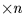(columns)                       matrix, where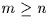x 		 is the n-vector we wish to find, and
b 		 is a given m-vector

by means of the Singular Value Decomposition method (SVD).

CALL:
CALL sla_SVDSOL (M, N, MP, NP, B, U, W, V, WORK, X)

GIVEN:

 I m, n, the numbers of rows and columns in matrix A M,N MP,NP I physical dimensions of array containing matrix A B D(M) known vector b U D(MP,NP) array containing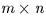matrix U W D(N)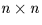diagonal matrix W (diagonal elements only) V D(NP,NP) array containingorthogonal matrix V

RETURNED:

 D(N) WORK workspace X D(N) unknown vector x

NOTES:
1.
In the Singular Value Decomposition method (SVD), the matrix A is first factorized (for example by the routine sla_SVD) into the following components:

A = UWVT

where:

A 		 is any m (rows)(columns) matrix,                      where m > n
U 		 is ancolumn-orthogonal matrix
W 		 is andiagonal matrix with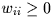VT is the transpose of anorthogonal matrix

Note that m and n are the logical dimensions of the matrices and vectors concerned, which can be located in arrays of larger physical dimensions MP and NP. The solution is then found from the expression:

x = V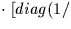W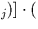U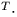b)

2.
If matrix A is square, and if the diagonal matrix W is not altered, the method is equivalent to conventional solution of simultaneous equations.
3.
If m > n, the result is a least-squares fit.
4.
If the solution is poorly determined, this shows up in the SVD factorization as very small or zero Wj values. Where a Wj value is small but non-zero it can be set to zero to avoid ill effects. The present routine detects such zero Wj values and produces a sensible solution, with highly correlated terms kept under control rather than being allowed to elope to infinity, and with meaningful values for the other terms.

REFERENCE:
Numerical Recipes, section 2.9.Next: SLA_TP2S - Tangent Plane to Spherical
Up: SUBPROGRAM SPECIFICATIONS
Previous: SLA_SVDCOV - Covariance Matrix from SVD

SLALIB --- Positional Astronomy Library